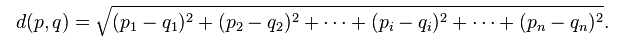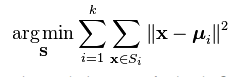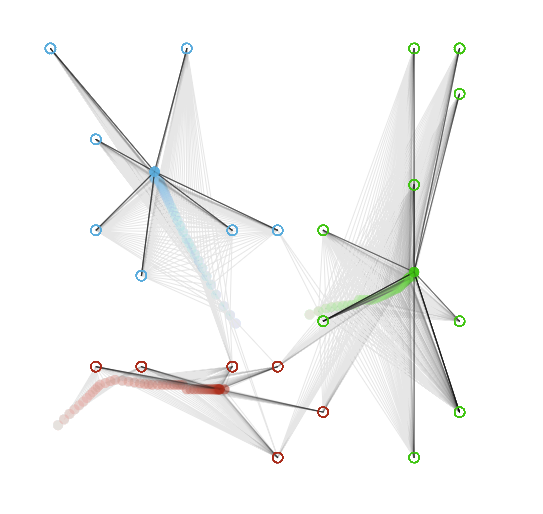# K-Means Clustering in JavaScript

Clustering is grouping of data or dividing a large data set into smaller data sets of some similarity. A well known clustering algorithm in unsupervised machine learning is K-Means clustering. The K-Means algorithm takes in `n` observations (data points), and groups them into `k` clusters, where each observation belongs to a cluster based on the nearest mean (cluster centroid). The distance between a data point and cluster centroid is calculated using the Euclidean distance.# Procedure

How the K-Means algorithm works is relatively straight forward. We just follow these steps:

• Step 1. Plot data points.
• Step 2. Initialize `k` additional points which are the seeds (cluster centroids) by plotting them randomly on the graph within the boundaries of the `n` observation’s dimenion ranges.
• Step 3. Assign each `n` data point to it’s closest cluster centroid (mean).
• Step 4. Move the cluster centroid to the average position of all the data points that belong to that mean.
• Step 5: Repeat steps 3 and 4 until there is no change in each cluster centroid movement. If means remain the same (nothing moved) then we know the algorithm has finished.

`K` is usually found by another algorithm to achieve the best k value, for example, by using the Bayesian information criterion, The Elbow Method, or the Rule of thumb, which is simply `k= √(n/2)`.

# AlgorithmGiven a set of observations `(x1, x2, …, xn)`, where each observation is a d-dimensional real vector, k-means clustering aims to partition the n observations into `k (≤ n)` sets `S = {S1, S2, …, Sk}` so as to minimize the within-cluster sum of squares (WCSS) where `μi` is the mean of points in `Si`. (wikipedia)

### Credit

Most of my understanding and knowledge of the K-Means algorithm and implementation came from this article by Burak Kanber.

``````/**
* KMeans
* @constructor
* @desc KMeans constructor
* @param {object} options - options object
* @param {object} options.canvas - canvas element
* @param {array} options.data - data array with points
* @param {number} options.k - number of cluster centroids
* @return array with arrays of points
*/
function KMeans(opts) {
// Create new instance if `new` keyword was not used.
if (!(this instanceof KMeans)) {
return new KMeans(opts);
}

opts = opts || {};
this.canvas = opts.canvas;
this.context = this.canvas.getContext('2d');
this.width = this.canvas.width;
this.height = this.canvas.height;

// Number of cluster centroids.
this.k = opts.k;

// Points to cluster.
this.data = opts.data;

// Keeps track of which cluster centroid index each data point belongs to.
this.assignments = [];

// Get the extents (min,max) for the dimensions.

// Get the range of the dimensions.
this.ranges = this.dataExtentRanges();

// Generate random cluster centroid points.
this.means = this.seeds();

// Generate cluster colors.
this.clusterColors = this.clusterColors();

// Keep track of number of times centroids move.
this.iterations = 0;

// Clear the canvas.
this.context.fillStyle = 'rgb(255,255,255)';
this.context.fillRect(0, 0, this.width, this.height);

// Draw the points onto canvas.
this.draw();

// Delay for each draw iteration.
this.drawDelay = 20;

// Perform work.
this.run();
}

/**
* @desc Returns the the minimum and maximum values for each dimention in the data array.
* @param {array} data - data containing points
* @return {array} extents - extents for each dimenion
* @example
* kmeans.data = [
*   [2,5],
*   [4,7],
*   [3,1]
* ];
* var extents = kmeans.dataDimensionExtents();
* console.log(extents); // [{min: 2, max: 4}, {min: 1, max: 7}]
*/
KMeans.prototype.dataDimensionExtents = function() {
data = data || this.data;
var extents = [];

for (var i = 0; i < data.length; i++) {
var point = data[i];

for (var j = 0; j < point.length; j++) {
if (!extents[j]) {
extents[j] = {min: 1000, max: 0};
}

if (point[j] < extents[j].min) {
extents[j].min = point[j];
}

if (point[j] > extents[j].max) {
extents[j].max = point[j];
}
}
}

return extents;
};

/**
* dataExtentRanges
* @desc Returns the range for each extent
* @return {array} ranges
* kmeans.extents = [{min: 2, max: 4}, {min: 1, max: 7}]
* var ranges = kmeans.dataExtentRanges(extents);
* console.log(ranges); // [2,6]
*/
KMeans.prototype.dataExtentRanges = function() {
var ranges = [];

for (var i = 0; i < this.extents.length; i++) {
ranges[i] = this.extents[i].max - this.extents[i].min;
}

return ranges;
};

/**
* seeds
* @desc Returns an array of randomly generated cluster centroid points bounds based on the data dimension ranges.
* @return {array} cluster centroid points
* @example
* var means = kmeans.seeds();
* console.log(means); // [[2,3],[4,5],[5,2]]
*/
KMeans.prototype.seeds = function() {
var means = [];
while (this.k--) {
var mean = [];

for (var i = 0; i < this.extents.length; i++) {
mean[i] = this.extents[i].min + (Math.random() * this.ranges[i]);
}

means.push(mean);
}

return means;
};

/**
* assignClusterToDataPoints
* @desc Calculate Euclidean distance between each point and the cluster center.
* Assigns each point to closest mean point.
*
* The distance between two points is the length of the path connecting them.
* The distance between points P(p1,p2) and Q(q1,q2) is given by the Pythagorean theorem.
*
* distance = square root of ((p1 - q1)^2 + (p2 - q2)^2)
*
* For n dimensions, ie P(p1,p2,pn) and Q(q1,q2,qn).
* d(p,q) = square root of ((p1 - q1)^2 + (p2 - q2)^2 + ... + (pn - qn)^2)
*
* http://en.wikipedia.org/wiki/Euclidean_distance
*/
KMeans.prototype.assignClusterToDataPoints = function() {
var assignments = [];

for (var i = 0; i < this.data.length; i++) {
var point = this.data[i];
var distances = [];

for (var j = 0; j < this.means.length; j++) {
var mean = this.means[j];
var sum = 0;

/* We calculate the Euclidean distance.
* √((pi-qi)^2+...+(pn-qn)^2)
*/

for (var dim = 0; dim < point.length; dim++) {
// dif = (pn - qn)
var difference = point[dim] - mean[dim];

// dif = (dif)^2
difference = Math.pow(difference, 2);

// sum = (difi) + ... + (difn)
sum += difference;
}

// √sum
distances[j] = Math.sqrt(sum);
}

// After calculating all the distances from the data point to each cluster centroid,
// we pick the closest (smallest) distances.
assignments[i] = distances.indexOf(Math.min.apply(null, distances));
}

return assignments;
};

/**
* moveMeans
* @desc Update the positions of the the cluster centroids (means) to the average positions
* of all data points that belong to that mean.
*/
KMeans.prototype.moveMeans = function() {
var sums = fillArray(this.means.length, 0);
var counts = fillArray(this.means.length, 0);
var moved = false;
var i;
var meanIndex;
var dim;

// Clear location sums for each dimension.
for (i = 0; i < this.means.length; i++) {
sums[i] = fillArray(this.means[i].length, 0);
}

// For each cluster, get sum of point coordinates in every dimension.
for (var pointIndex = 0; pointIndex < this.assignments.length; pointIndex++) {
meanIndex = this.assignments[pointIndex];
var point = this.data[pointIndex];
var mean = this.means[meanIndex];

counts[meanIndex]++;

for (dim = 0; dim < mean.length; dim++) {
sums[meanIndex][dim] += point[dim];
}
}

/* If cluster centroid (mean) is not longer assigned to any points,
* move it somewhere else randomly within range of points.
*/
for (meanIndex = 0; meanIndex < sums.length; meanIndex++) {
if (0 === counts[meanIndex]) {
sums[meanIndex] = this.means[meanIndex];

for (dim = 0; dim < this.extents.length; dim++) {
sums[meanIndex][dim] = this.extents[dim].min + (Math.random() * this.ranges[dim]);
}
continue;
}

for (dim = 0; dim < sums[meanIndex].length; dim++) {
sums[meanIndex][dim] /= counts[meanIndex];
sums[meanIndex][dim] = Math.round(100*sums[meanIndex][dim])/100;
}
}

/* If current means does not equal to new means, then
* move cluster centroid closer to average point.
*/
if (this.means.toString() !== sums.toString()) {
var diff;
moved = true;

// Nudge means 1/nth of the way toward average point.
for (meanIndex = 0; meanIndex < sums.length; meanIndex++) {
for (dim = 0; dim < sums[meanIndex].length; dim++) {
diff = (sums[meanIndex][dim] - this.means[meanIndex][dim]);
if (Math.abs(diff) > 0.1) {
var stepsPerIteration = 10;
this.means[meanIndex][dim] += diff / stepsPerIteration;
this.means[meanIndex][dim] = Math.round(100*this.means[meanIndex][dim])/100;
} else {
this.means[meanIndex][dim] = sums[meanIndex][dim];
}
}
}
}

return moved;
};

/**
* run
* @desc Reassigns nearest cluster centroids (means) to data points,
* and checks if cluster centroids (means) have moved, otherwise
* end program.
*/
KMeans.prototype.run = function() {
++this.iterations;

// Reassign points to nearest cluster centroids.
this.assignments = this.assignClusterToDataPoints();

// Returns true if the cluster centroids have moved location since the last iteration.
var meansMoved = this.moveMeans();

/* If cluster centroids moved then
*rerun to reassign points to new cluster centroid (means) positions.
*/
if (meansMoved) {
this.draw();
this.timer = setTimeout(this.run.bind(this), this.drawDelay);
} else {
// Otherwise task has completed.
console.log('Iteration took for completion: ' + this.iterations);
}
};

KMeans.prototype.draw = function() {
// Slightly clear the canvas to make new draws visible.
this.context.fillStyle = 'rgba(255,255,255, 0.2)';
this.context.fillRect(0, 0, this.width, this.height);

var point;
var i;

/* Iterate though points draw line from their origin to their cluster centroid.
* `assignments` contains cluster centroid index for each point.
*/
for (i = 0; i < this.assignments.length; i++) {
var meanIndex = this.assignments[i];
point = this.data[i];
var mean = this.means[meanIndex];

// Make lines that will get drawn alpha transparent.
this.context.globalAlpha = 0.1;

// Push current state onto the stack.
this.context.save();

this.context.beginPath();

// Begin path from current point origin.
this.context.moveTo(
(point - this.extents.min + 1) * (this.width / (this.ranges + 2)),
(point - this.extents.min + 1) * (this.height / (this.ranges + 2))
);

// Draw path from the point (moveTo) to the cluster centroid.
this.context.lineTo(
(mean - this.extents.min + 1) * (this.width / (this.ranges + 2)),
(mean - this.extents.min + 1) * (this.height / (this.ranges + 2))
);

// Draw a stroke on the path to make it visible.
this.context.strokeStyle = 'black';
this.context.stroke();
//this.context.closePath();

// Restore saved state.
this.context.restore();
}

// Plot every point onto canvas.
for (i = 0; i < data.length; i++) {
this.context.save();

point = this.data[i];

// Make style fully opaque.
this.context.globalAlpha = 1;

// Move canvas origin on the grid to current point position.
this.context.translate(
(point - this.extents.min + 1) * (this.width / (this.ranges + 2)),
(point - this.extents.min + 1) * (this.width / (this.ranges + 2))
);

this.context.beginPath();

// Draw point circle.
this.context.arc(0, 0, 5, 0, Math.PI*2, true);

// Set the color for current point based on which cluster it belongs to.
this.context.strokeStyle = this.clusterColor(this.assignments[i]);

// Draw a stroke to make circle visible.
this.context.stroke();
this.context.closePath();

this.context.restore();
}

// Draw cluster centroids (means).
for (i = 0; i < this.means.length; i++) {
this.context.save();

point = this.means[i];

this.context.globalAlpha = 0.5;
this.context.fillStyle = this.clusterColor(i);
this.context.translate(
(point - this.extents.min + 1) * (this.width / (this.ranges + 2)),
(point - this.extents.min + 1) * (this.width / (this.ranges + 2))
);
this.context.beginPath();
this.context.arc(0, 0, 5, 0, Math.PI*2, true);
this.context.fill();
this.context.closePath();

this.context.restore();
}
};

/**
* clusterColors
* @desc Generate a random colors for clusters.
* @return random colors
*/
KMeans.prototype.clusterColors = function() {
var colors = [];

// Generate point color for each cluster.
for (var i = 0; i < this.data.length; i++) {
colors.push('#'+((Math.random()*(1<<24))|0).toString(16));
}

return colors;
};

/**
* clusterColor
* @desc Get color for cluster.
* @param {number} index - cluster (mean) index
* @return color for cluster
*/
KMeans.prototype.clusterColor = function(n) {
return this.clusterColors[n];
};

/**
* fillArray
* @desc Returns a prefilled array.
* @param {number} length - length of array
* @param {*} value - value to prefill with.
* @return array with prefilled values.
*/
function fillArray(length, val) {
return Array.apply(null, Array(length)).map(function() { return val; });
}
``````

# Usage

Let’s see it in action.

``````var data = [
[6,5],
[9,10],
[10,1],
[5,5],
[7,7],
[4,1],
[10,7],
[6,8],
[10,2],
[9,4],
[2,5],
[9,1],
[10,9],
[2,8],
[1,1],
[6,10],
[3,8],
[2,3],
[7,9],
[7,7],
[3,6],
[5,8],
[7,5],
[10,9],
[10,9]
];

var kmeans = new KMeans({
canvas: document.getElementById('canvas'),
data: data,
k: 3
});
``````View the demo »

# Conclusion

As you can see in the demo, K-Means does a fairly good job in clustering data. If you run the algorithm thousands of times you can determine the best solution based on which solutions got returned the most often. Sometimes your dataset contains outliers and the mean may not be the best measure for clustering because the clusters may be heavily skewed, so in this scenario the K-Medians algorithm is your best bet.